# RGB LED bulb control | Step by step instructions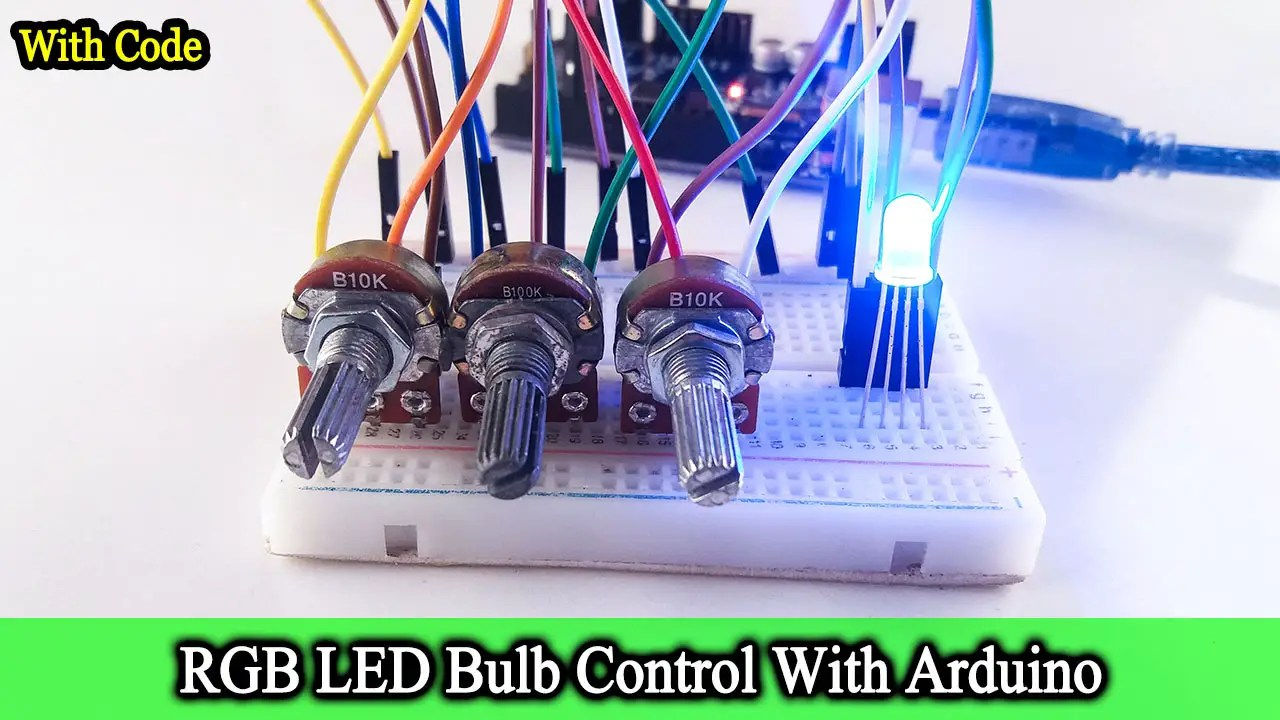Hello guys, Welcome back to my SriTu Hobby blog. Today we will learn how to control an RGB LED bulb. Let us first see what an RGB LED bulb is. An RGB LED Bulb is a diode made up of three colors: red, green, and blue. We can get the desired color by adding or subtracting the three colors here. Also, we can light these three colors separately. We can usually see two pins of an LED bulb, but in this RGB LED bulb, we can see four pins. Here we can identify three pins as the anode terminal of the three colors and the other pin as the cathode terminal of the three colors. In particular, the cathode pin is longer than the other pins.

We will now test this RGB LED bulb using an Arduino board. For this, we need three Potentiometers in addition to the RGB bulb. The potentiometer is a variable resistor. Here the resistance value is changed by twisting the knob. Through these, we can increase or decrease the brightness of the three diodes in the RGB LED bulb.

Well now let’s see what components are needed for this project. You can easily buy these components using the links below.

Ok, let’s do this project step by step.

### Step 1

First, identify these components.
Arduino UNO board.You can use any other Arduino board.
RGB LED bulb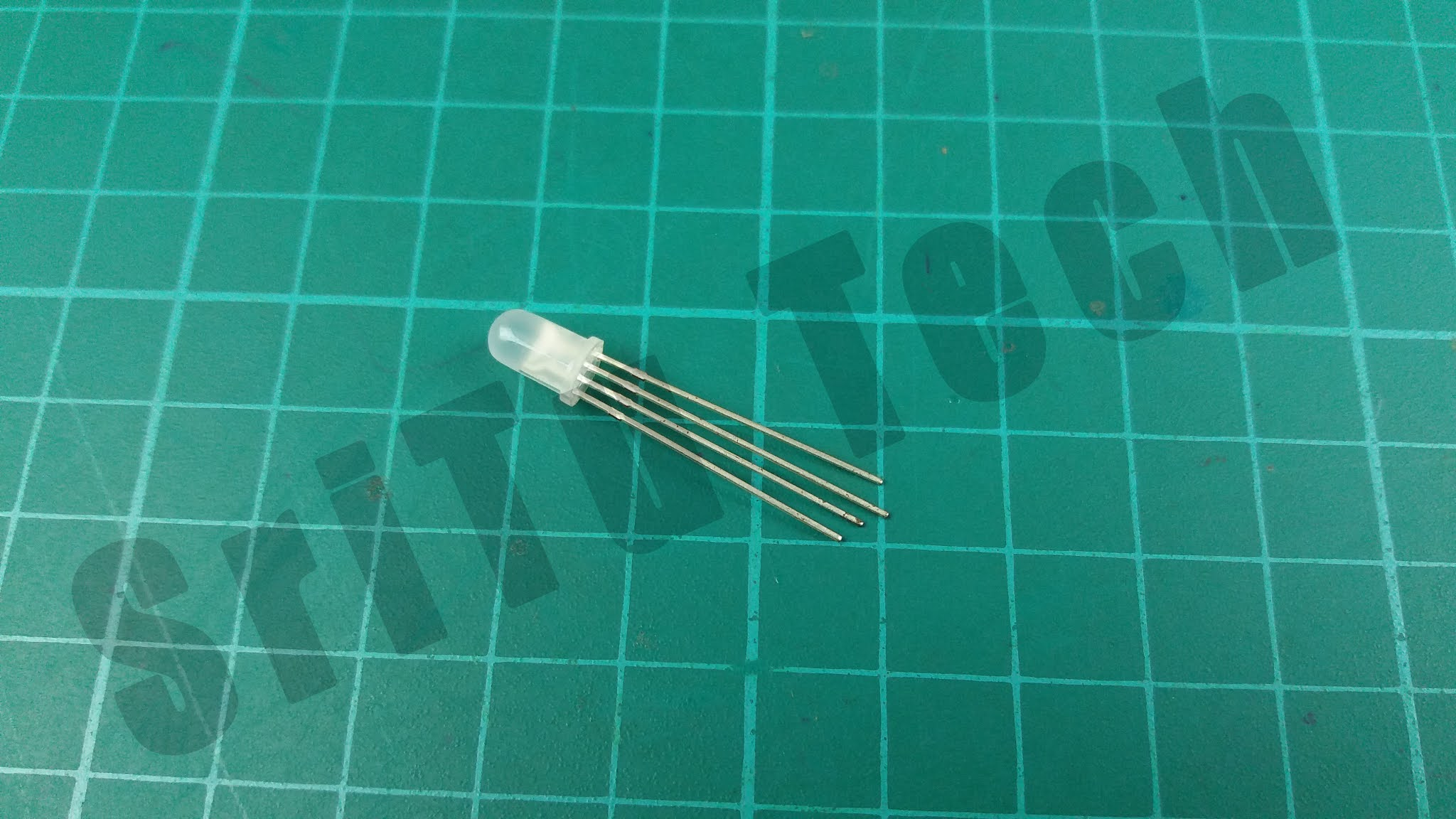Described above.
Potentiometer180-ohm resistor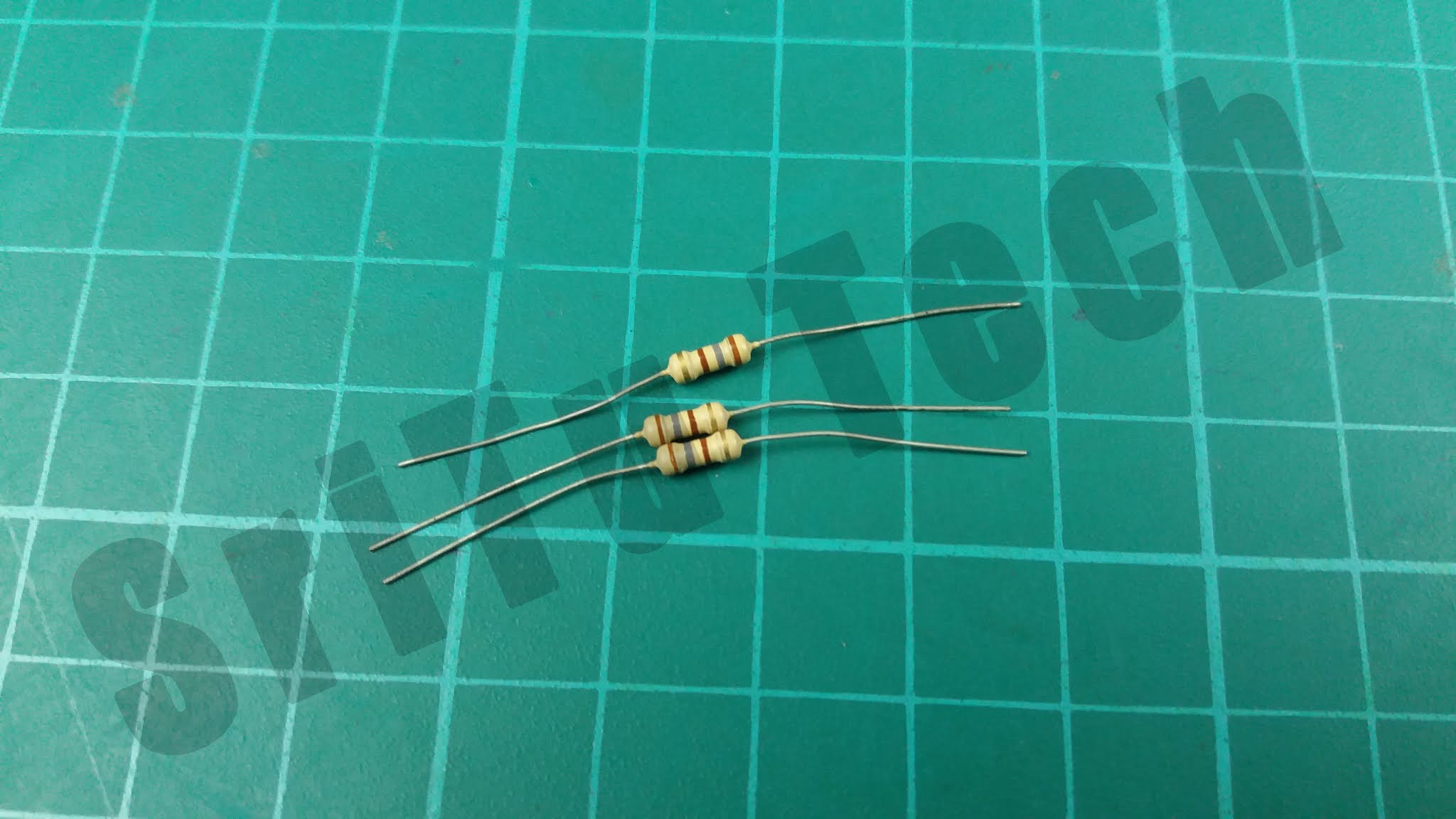This component is using controlling the resistance.Jumper wires### Step 2

Now connect these components. For that, using the circuit diagram below.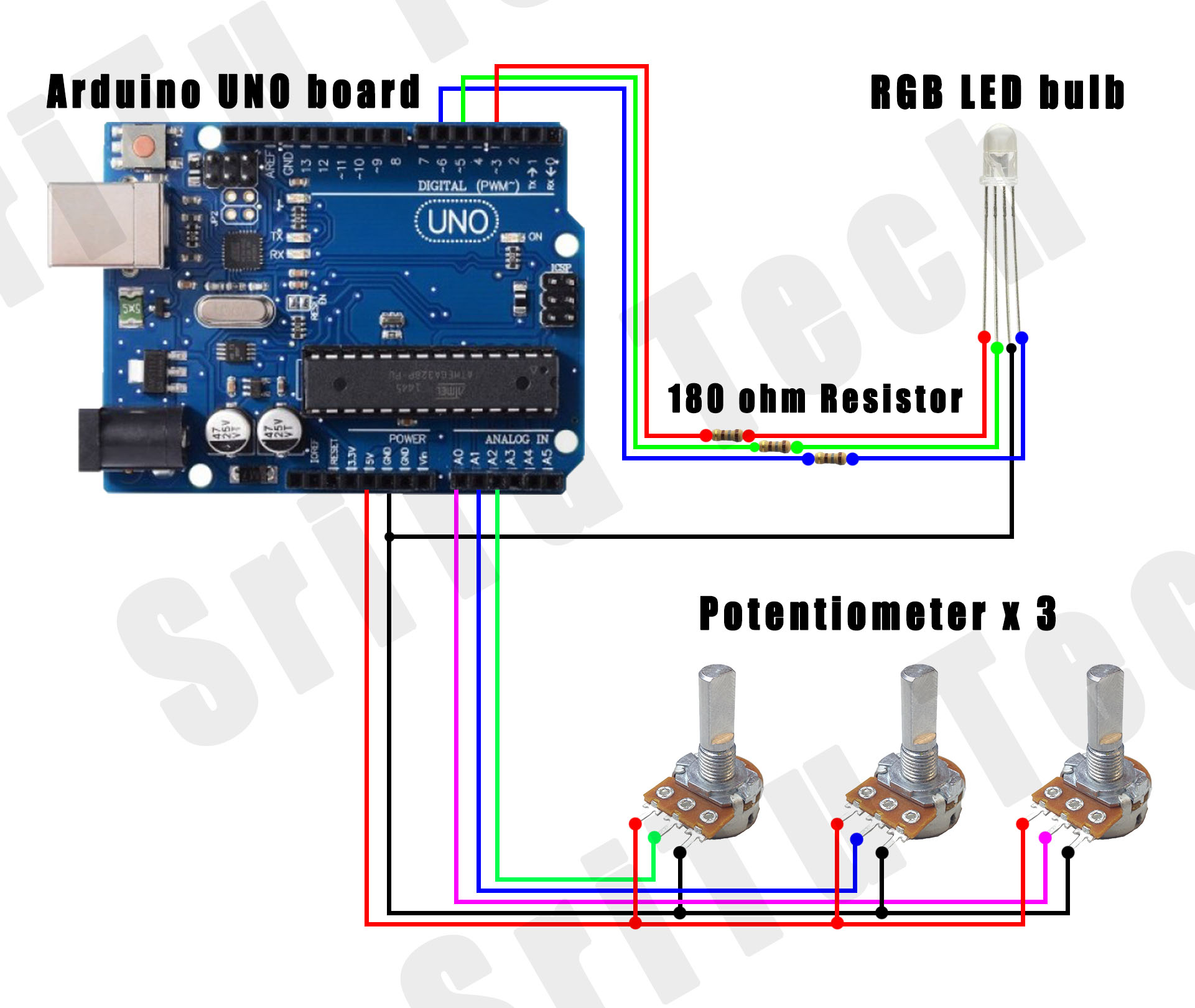### Step 3

Well now let’s look at the program required for this. You can see this program below.
The complete program of this project – Download
``````/*RGB LED bulb PWM with Arduino.
created by the SriTu Tech team.
Read the code below and use it for any of your creation
*/
#define red 3
#define green 5
#define blue 6

void setup() {
Serial.begin(9600);
pinMode(red, OUTPUT);
pinMode(green, OUTPUT);
pinMode(blue, OUTPUT);
}

void loop() {
int p1 = analogRead(A0);
int p2 = analogRead(A1);
int p3 = analogRead(A2);

p1 = map(p1, 0, 1023, 0, 255);
analogWrite(red, p1);
Serial.println(p1);
p2 = map(p2, 0, 1023, 0, 255);
analogWrite(green, p2);
Serial.println(p2);
p3 = map(p3, 0, 1023, 0, 255);
analogWrite(blue, p3);
Serial.println(p3);
}``````

#### Code explanation

First, the Arduino pins connected to the anode terminals of the RGB LED bulb are defined. These are PWM pins.
#define red 3
#define green 5
#define blue 6

Later, in this Void setup, these pins are converted to output pins.
void setup() {
Serial.begin(9600);
pinMode(red, OUTPUT);
pinMode(green, OUTPUT);
pinMode(blue, OUTPUT);
}

Next, the value from 0 to 1024 received by the Potentiometer is changed from 0 to 255 and that value is written on the PWM pin connected to the tip of the RGB LED bulb. This is inserted in the Void loop.
void loop() {
int p1 = analogRead(A0);
int p2 = analogRead(A1);
int p3 = analogRead(A2);

p1 = map(p1, 0, 1023, 0, 255);
analogWrite(red, p1);
Serial.println(p1);
p2 = map(p2, 0, 1023, 0, 255);
analogWrite(green, p2);
Serial.println(p2);
p3 = map(p3, 0, 1023, 0, 255);
analogWrite(blue, p3);
Serial.println(p3);
}

### Step 4

Now select the correct board and port. Finally, upload this code and enjoy it. Below is the full video guide.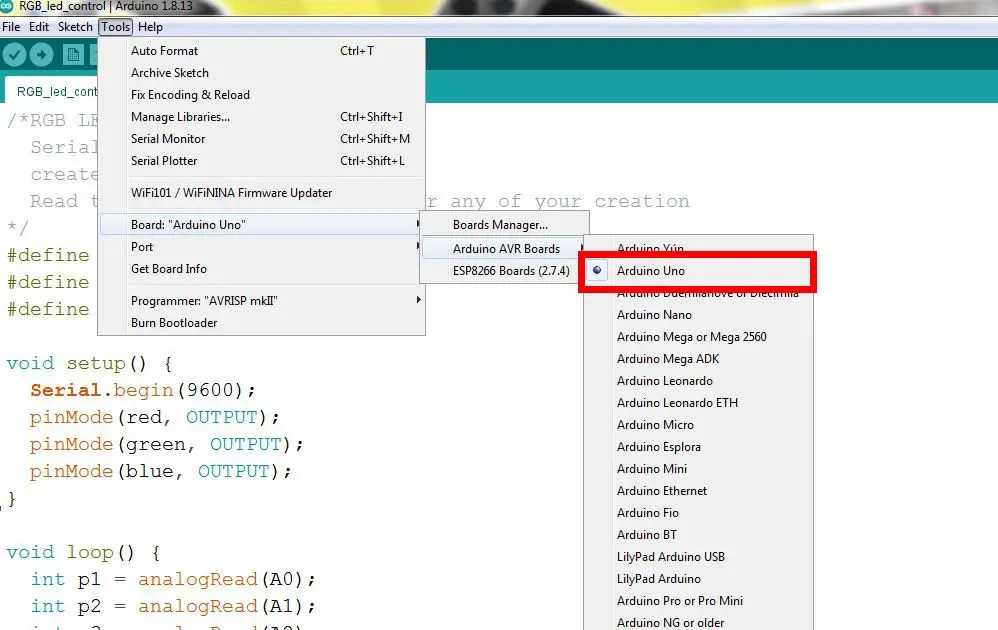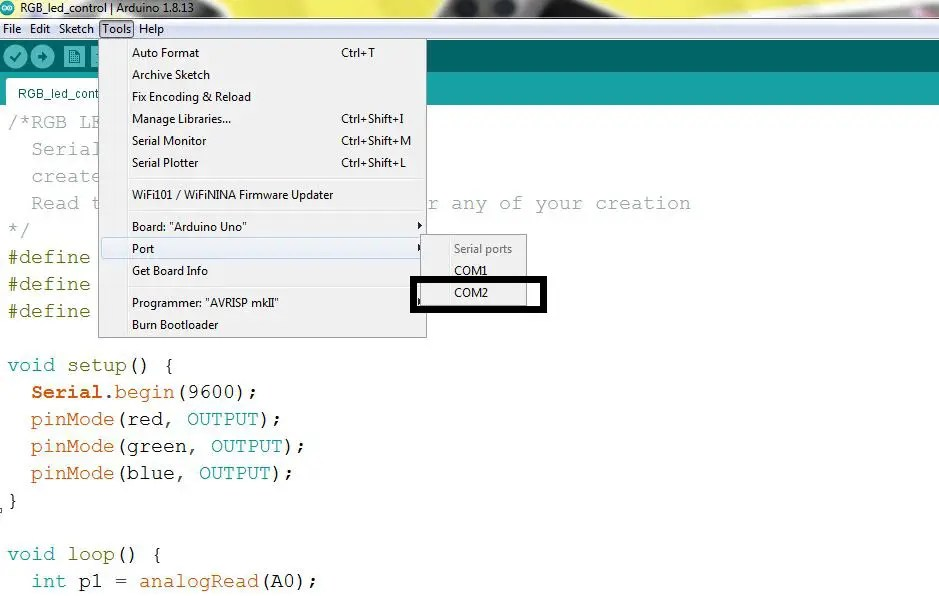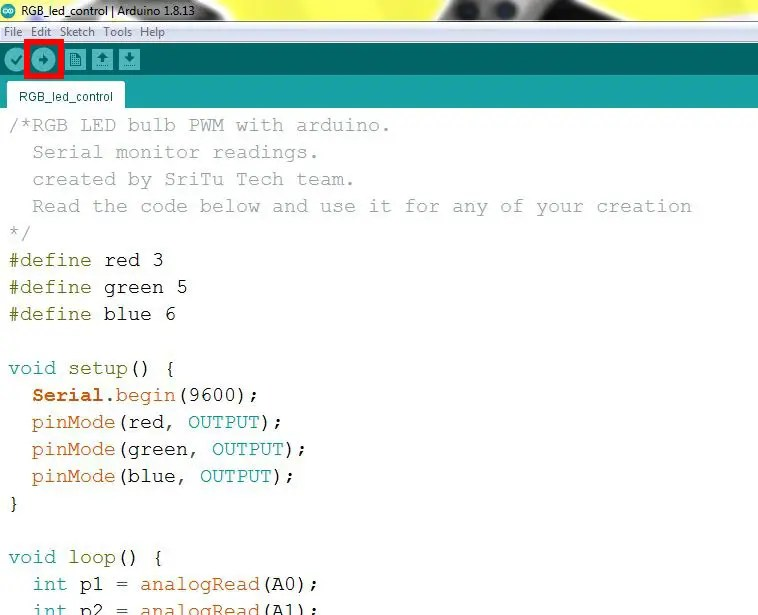I think you may have learned something from this article. We will meet in the next article. Bye-bye.